# Recursive Tree Traversals of a Binary Tree: Preorder, Inorder and Postorder Traversal

### Introduction to tree traversal

To process data stored in the tree data structure, we need to traverse each node of the tree. The process to visit all nodes of a tree is called tree traversal. It is just like searching the tree and access each node only once. During the visit of a tree node, we can perform various operations (query or modification) with the data stored in the node.

We access data elements in sequential order in the case of linear data structures (like linked lists, stacks, queues, etc.). But in binary tree data structures, there can be many different ways to access data elements. Starting from the root node, there are two directions to go — we can go left via the left pointer or we can go right via the right pointer. So what are possible options? Let’s Explore!

### Types of tree traversal

A binary tree is a recursive object where we have three essential components:

• root node: the topmost node of the binary tree
• left-subtree: a smaller binary tree connected via the left pointer of the root
• right-subtree: a smaller binary tree connected via the right pointer of the root

So traversing a binary tree requires each component's traversal: processing root node, traversing the left subtree, and traversing the right subtree.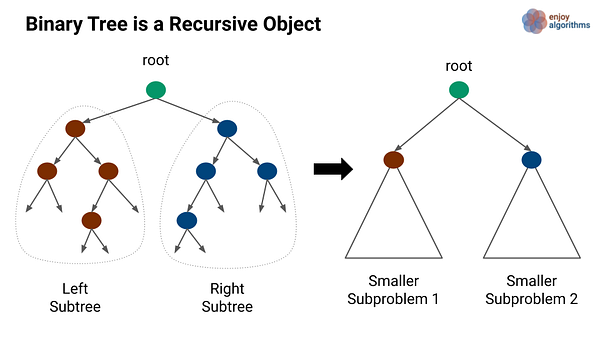If we look from another perspective, the left and right subtree are binary trees with smaller nodes and part of the same binary tree. So we can implement tree traversal easily using simple recursion i.e. by dividing a problem of binary tree traversal into traversal of root and the traversal of two smaller subproblems:

• Subproblem 1: Traversal of the left subtree
• Subproblem 2: Traversal of the right subtree

Here is another insight: there can be various choices to traverse each component, where each choice traverses nodes in a different order and provide a different traversal. So the classification of traversal is based on the order in which the nodes are visited: 1) first visit the root then traverse left or right subtree 2) first traverse either left or right subtree then visit root 3) first traverse left and right subtree and visit root in the last. In other words, we have 3! or 6 possibilities where each possibility will process the nodes in a different order.

• Preorder: visit root → Traverse left subtree → Traverse right subtree
• Reverse Preorder: visit root → Traverse right subtree → Traverse left subtree
• Inorder: Traverse left subtree → visit root → Traverse right subtree
• Reverse Inorder: Traverse right subtree → visit root → Traverse left subtree
• Postorder: Traverse left subtree → Traverse right subtree → visit root
• Reverse Postorder: Traverse left subtree → Traverse right subtree → visit root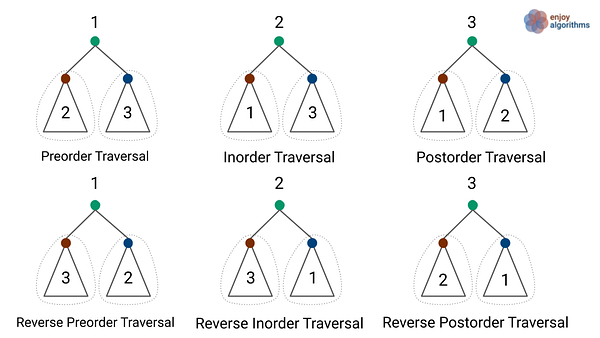If further simplify the classification based on the order in which we visit the root, then it would get reduced to three traversals: preorder(root first), inorder (root second) and postorder(root third). These traversals are also called the DFS traversal of a binary tree. Note: All three reverse traversals are the mirror image of these traversals. Sometimes reverse traversals also help us to solve several coding problems in a binary tree.

There is another traversal method where we visit nodes in a level-wise order. This is called level order traversal or breadth-first search traversal (BFS traversal). Here we will discuss the idea of recursive DFS traversals. We have separately discussed the Iterative DFS traversal using stack.

### Recursive preorder traversal of a binary tree

In this traversal, we first process the data stored in the root node, then we process all nodes in the left subtree recursively, and finally process all the nodes in the right subtree recursively. Suppose we are using a function preorder(root) with root as an input parameter. Here are the simple steps of recursive implementation:

• First, process the data stored in the root node i.e. process(root->value). We can do various data manipulation operations like printing the node data, arithmetic operations, logical operations, etc.
• Then we recursively traverse and process each node in the left subtree by calling the same function with root->left as input parameter i.e. preorder(root->left).
• Then we recursively traverse and process each node in the right subtree by calling the same function with root->right as input parameter i.e. preorder(root->right).
• Base case: recursion will terminate and backtrack when it reaches the bottommost end of the binary tree, i.e. root == NULL.

Pseudocode of recursive preorder traversal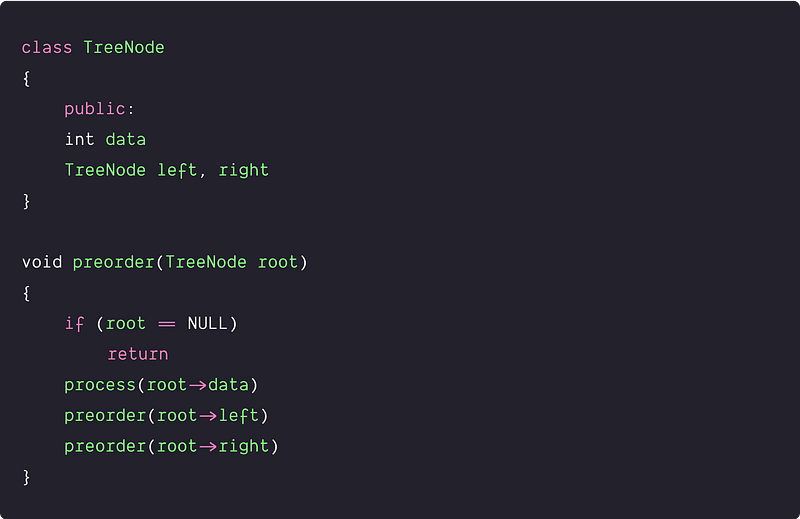Visualization of the recursive preorder traversal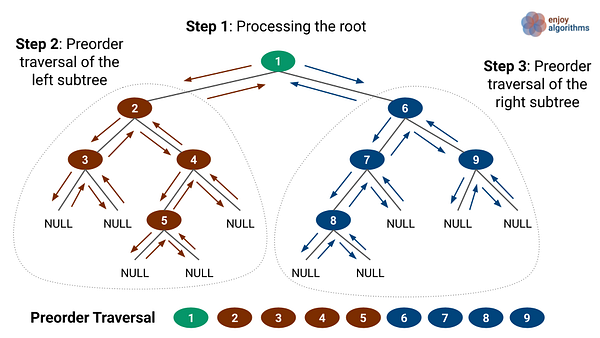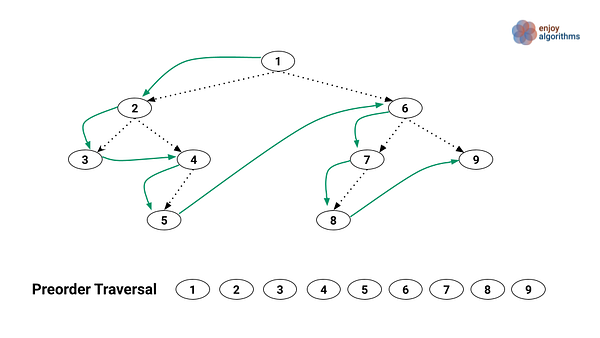Application of Preorder Traversal

We use preorder traversal in a situation when we need to explore all the tree nodes before inspecting any leaves.

• To create a replica or copy of a tree.
• To get the prefix expression of an expression tree.

### Recursive inorder traversal of a binary tree

In this traversal, we first process all nodes in the left subtree recursively, process the data stored in the root node, finally process all the nodes in the right subtree recursively. Suppose we are using a function inorder(root) with root as an input parameter. Here are the simple steps to recursive implementation:

• Before processing the root node, we recursively traverse and process each node in the left subtree by calling the same function with root->left as input parameter i.e. inorder(root->left).
• After processing each node in the left subtree, now we process data stored in the root node i.e. process(root->data).
• Finally, we recursively traverse and process each node in the right subtree by calling the same function with root->right as input parameter i.e. inorder(root->right).
• Base case: recursion will terminate and backtrack when it reaches the end of the tree i.e. root == NULL.

Pseudocode of recursive Inorder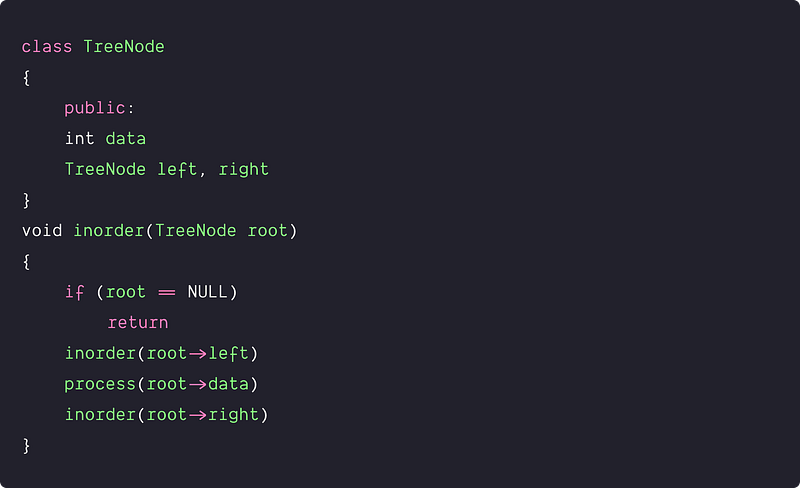Visualisation of the Inorder traversal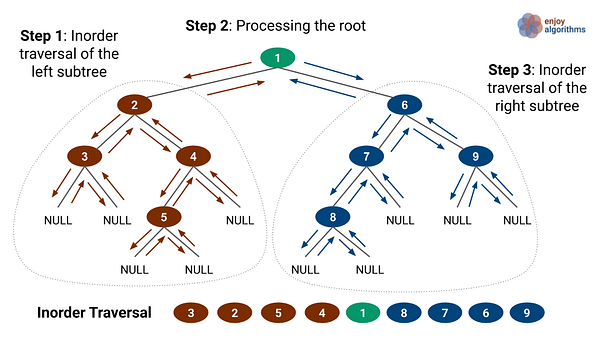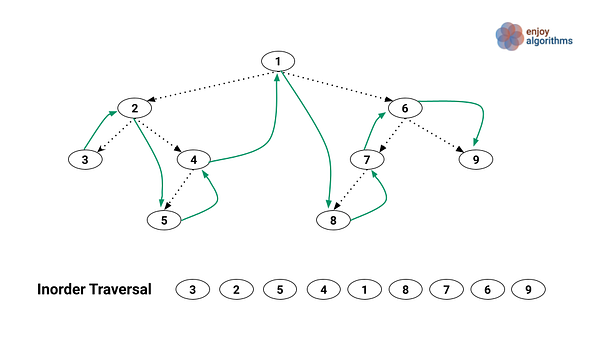Application of Inorder Traversal

It is commonly used in the processing of binary search trees. In BST, there is an order associated with each node: all values in the left subtree ≤ node value ≤ all values in the right subtree. So inorder traversal of BST generates the node's data in increasing order.

### Recursive postorder traversal of a binary tree

Suppose we are using a function postorder(root) with root as an input parameter. We first process all the nodes in the left subtree, then process all the nodes in the right subtree and at the end, we process the root node.

• We first recursively traverse and process each node in the left subtree by calling the same function with root->left as input parameter i.e. postorder(root->left).
• Then we recursively traverse and process each node in the right subtree by calling the same function with root->right as input parameter i.e. inorder(root->right).
• Finally, we process the data stored in the root node i.e. process(root->value).
• Base case: recursion will terminate and backtrack when it reaches the bottommost end of the tree i.e. root == NULL.

Pseudocode of recursive postorder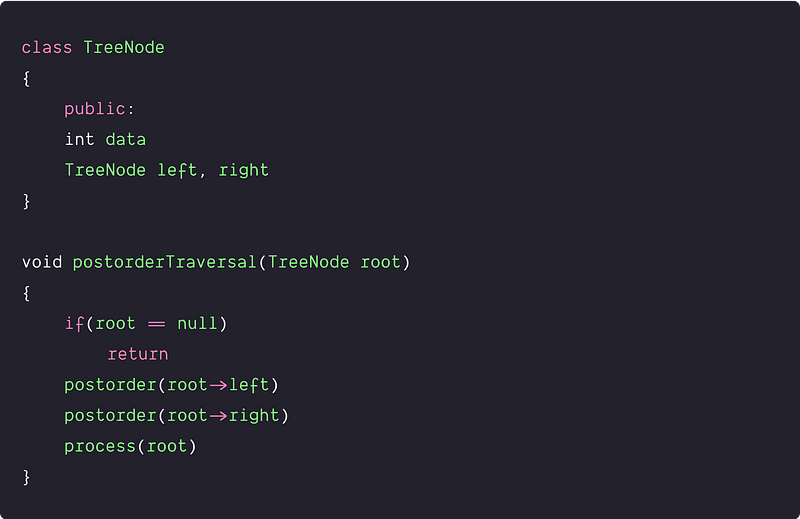Visualisation of the postorder traversal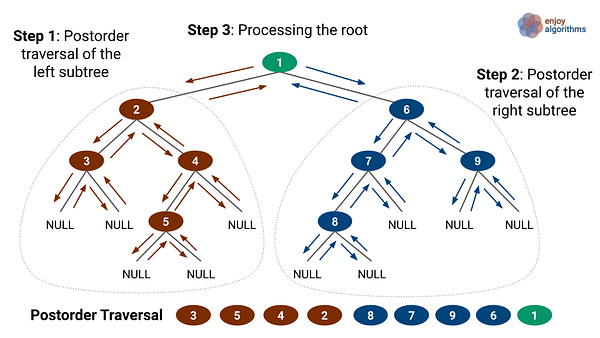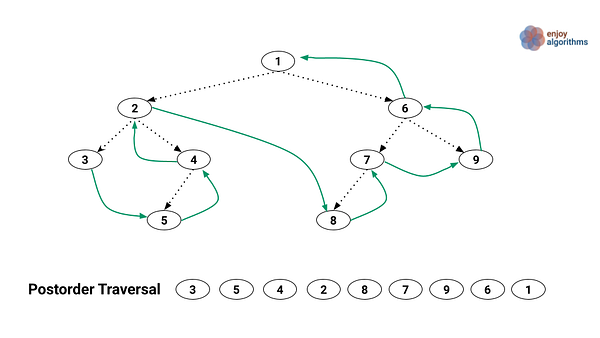Application of Postorder Traversal

We use postorder traversal when we need to explore all the leaf nodes before inspecting any internal nodes.

• Deleting the entire binary tree
• To get postfix expression on of an expression tree.

### Time complexity analysis of recursive DFS Traversals

One simple idea for all the DFS traversal would be: accessing each node once and doing constant operation with each node. So time complexity = number of nodes * operation with each node = n*O(1) = O(n)

But let’s visualize it by following the analysis process of the recursive algorithms. In all the DFS traversals, we have two different subproblems :  left subtree and right subtree. The size of these subproblems depends on the number of nodes present in both subtrees. Suppose:

• T(n) is the time complexity of the traversal of n size tree
• k nodes are present in the left subtree and n  -  k  -  1 node on the right subtree. Here 0≤ k ≤ n — 1.
• We are doing an O(1) operation in processing any node.

T(n) = Time complexity of traversing left subtree + Time complexity of traversing right subtree + Time complexity of processing root node = T(k) + T(n — k — 1) + O(1) = T(k) + T(n — k — 1) + c

Recurrence relation T(n) = T(k) + T(n - k - 1) + c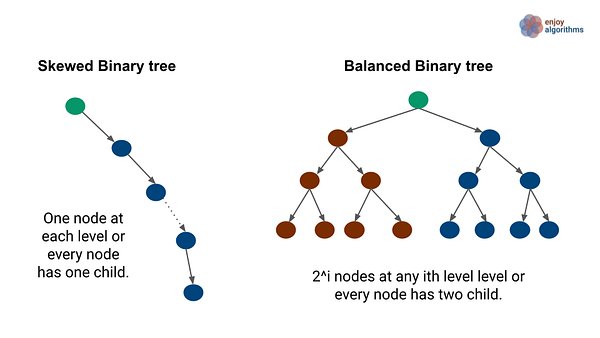Case 1: Skewed binary tree

In this situation, one subtree is empty and other subtree is non-empty. This will be the case of k = 0 or k = n — 1. Recurrence relation: T(n) = T(0) + T(n - 1) + c. Let's assume T(0) will be some constant because traversing an empty tree will take some constants time => T(0) + c = d (some constant)

Final recurrence relation: T(n) = T(n - 1) + d. Now we can easily solve this recurrence by expanding each intermediate value of T(n-i), for 1<= i <= n-1.

``````T(n) = T(n - 1) + d
T(n) = T(n-2) + d + d
T(n) = T(n-3) + d + d + d
and so on ...
T(n) = T(1) + (n-1)d
T(n) = T(0) + nd = O(n)
``````

Case 2: Balanced binary tree

In this situation, both left and right subtrees have an equal number of nodes. This will the case of k = (n -1)/2.

``````T(k) = T((n - 1)/2)
T(n - k - 1) = T((n - 1)/2)
Recurrence relation: T(n) = 2T((n - 1)/2) + c
``````

Let's simplify it further and assume that T(n) is a monotonically increasing function. Then, 2T((n - 1)/2) < 2T(n/2). If we place 2T(n/2) in place of 2T((n - 1)/2) in the above equation, then it would give an upper bound on time complexity.

T(n) = 2T(n/2) + c. We can easily solve this recurrence using the master theorem or recursion tree method. T(n) = O(n) (Think!). Refer to this blog: Analysis of recursive algorithms.

So in both scenarios, the time complexity of DFS traversal is O(n).

### The space complexity of recursive DFS traversals

Since preorder, inorder and postorder traversals are depth-first search traversal and recursion is similar in nature. So the space complexity analysis for all DFS traversal will be similar in approach.

The space complexity of the DFS traversal depends on the size of the recursion call stack, which is equal to the maximum depth of the recursion or height of the tree(h). In other words, there will be at most h recursive calls on the stack at any given moment, and the system allocates recursion call of size O(h).

Best case: This is a scenario when the tree is balanced, and the height of the tree will be logn. So best-case space complexity of all the DFS traversal is O(logn).

Worst case: This is a scenario when the tree is highly unbalanced, and there is only one node at each level. This tree looks similar to a singly linked list. So the height of the tree is n, and the worst-case space complexity of all the DFS traversal is O(n).

### Critical ideas to think!

• Depth-first traversal starts from the root, goes to the depth as far as possible, and then backtracks from there. In other words, it visits nodes from the bottom of the tree. If we need to search for something in the depth of the tree or node closer to a leaf, we would prefer DFS.
• Sometimes we can solve tree coding problems using all the traversals, but in some cases, some specific traversal would be the only option that provides the best solution. Our goal should be to learn the use case of each depth-first search traversal in problem-solving.
• For solving binary tree problems, we perform various operations with each node during the traversal. Sometimes, we pass extra variables or pointers to the function parameters, use helper function, use parent pointers, store some extra data inside the node, and use data structures like the stack, queue, priority queue, etc.
• What would be the average case space complexity of the DFS traversal? Let's think!
• Understanding the order of nodes in each traversal is very important. For example, if we want to construct the binary tree from the given traversal, then one traversal is insufficient. We need at least two of the traversals i.e, preorder and postorder or preorder and inorder or postorder and inorder. Why? Think and explore.

### Critical concepts to explore further!

• Iterative DFS traversal using stack
• BFS Traversal of binary tree
• BFS vs DFS traversal comparison
• Properties and Types of binary tree
• Various binary tree operations like insertion, deletion, construction, height, merging, searching, and other transformation and query operations.
• Array-based implementation of the binary tree
• Properties and operations of Binary Search Tree

### Problems to solve using DFS traversals

• Left View of Binary Tree
• Maximum width of a binary tree
• Min Depth of Binary Tree
• Level with the maximum number of nodes
• Convert a binary tree into a mirror tree
• Find the diameter of a binary tree
• Print node at k distance from a given node
• Boundary Traversal of binary Tree
• Least Common Ancestor of two-node in Binary tree
• Construct binary tree from preorder and In-order Traversal

Please write comments if you find an error or you want to share more insights about the topic. Enjoy learning, Enjoy coding, Enjoy algorithms!

Get well-designed application and interview centirc content on ds-algorithms, machine learning, system design and oops. Content will be delivered weekly.